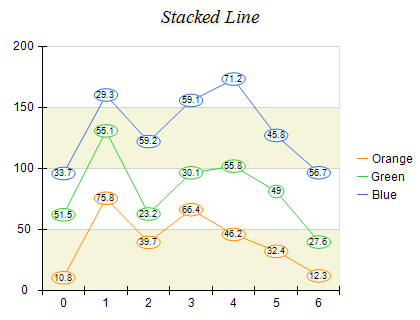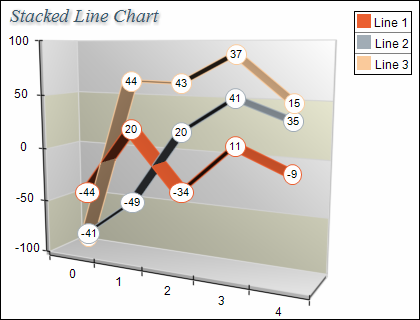﻿ Stacked Line
Nevron .NET Vision Documentation
Stacked Line

A Stacked Line chart displays related data groups, one on top of the other. It is used to show how each group contributes to the total as well as the trends of the total, usually over time. The individual series are displayed as sequences of straight line segments. Each line series is stacked on top of the previous one. The following figure displays a Stacked Line chart:Figure 1.

Creating the stacked line chart

Stacked Line charts are displayed with several NLineSeries objects. The MultiLineMode property of the first line series must be set to MultiLineMode.Series. The MultiLineMode property of the subsequent line series must be set to MultiLineMode.Stacked. The following example demonstrates how to create a stack line chart with two stacks:

C#
Copy Code
```// obtain a reference to the Cartesian chart that is created by default
NCartesianChart chart = (NCartesianChart)chartControl.Charts;

line1.MultiLineMode = MultiLineMode.Series;
line2.MultiLineMode = MultiLineMode.Stacked;
```
Visual Basic
Copy Code
```' obtain a reference to the Cartesian chart that is created by default
Dim chart As NCartesianChart = chartControl.Charts(0)

Dim line1 As NLineSeries = chart.Series.Add(SeriesType.Line)
Dim line2 As NLineSeries = chart.Series.Add(SeriesType.Line)

line1.MultiLineMode = MultiLineMode.Series
line2.MultiLineMode = MultiLineMode.Stacked
```

If you want to create a second stacked line with two stacks behind the stack line created in the previous sample you must add the following code:

C#
Copy Code
```NLineSeries line4 = (NLineSeries)chart.Series.Add(SeriesType.Line);

line4.MultiLineMode = MultiLineMode.Series;
line5.MultiLineMode = MultiLineMode.Stacked;
```
Visual Basic
Copy Code
```Dim line4 As NLineSeries = chart.Series.Add(SeriesType.Line)
Dim line5 As NLineSeries = chart.Series.Add(SeriesType.Line)

line4.MultiLineMode = MultiLineMode.Series
line5.MultiLineMode = MultiLineMode.Stacked
```
Stacks with negative values

Stacked line charts support negative values, which means that if you insert negative values in the Values data series the respective stacks will be piled below the zero. In this case since the values for a concrete category can be both positive and negative the chart will display two piles for each category - one for the positive values which grows above the zero and another one for the negative values which grows below the zero.

The following figure demonstrates a stack line chart with negative and positive values:figure 2.

Formatting Commands

The following formatting commands inherited from the NSeries class have different meaning when a line series is stacked:

<total> - displays the positive or negative values sum of the values in the current stack. If the current value is negative the negative sum is displayed otherwise the positive sum is displayed.
<cumulative> - displays the sum of the values up to the current stack value.
<percent> - displays the percent contribution of the value to the total pile sum.

Related Examples
Windows forms: Chart Gallery\Line\Stacked Line